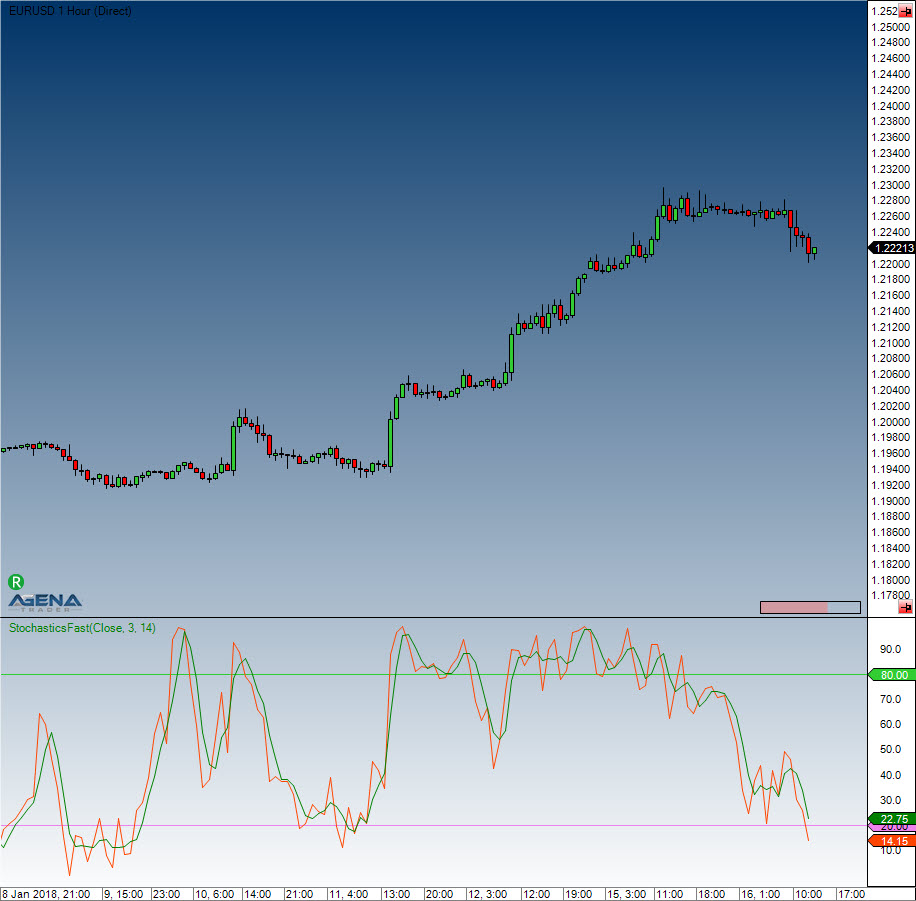Comment on page

# Stochastics Fast

#### Interpretation

Closing prices that are constantly in the upper area indicate accumulation (i.e. buying pressure), whilst the distribution (i.e. selling pressure) is shown by an indicator value in the lower area.

#### Usage

StochasticsFast(int periodD, int periodK)
StochasticsFast(IDataSeries inSeries, int periodD, int periodK)
//For the %D line
StochasticsFast(int periodD, int periodK).D[int barsAgo]
StochasticsFast(IDataSeries inSeries, int periodD, int periodK).D[int barsAgo]
//For the %K line
StochasticsFast(int periodD, int periodK).K[int barsAgo]
StochasticsFast(IDataSeries inSeries, int periodD, int periodK).K[int barsAgo]

#### Return value

double
When using this method with an index (e.g. StochasticsFast(7,14)[int barsAgo] ), the value of the indicator will be issued for the referenced bar.

#### Parameters

inSeries Input data series for the indicator
periodD Number of periods for the smoothing of %K
periodK Number of periods included in the calculation of %K

#### VisualizationStochastics Fast

#### Example

//Output for the %K line of the fast stochastic
Print("The stochastic line %K is currently at: " + StochasticsFast(3, 14).K);
//Output %D line
Print("The stochastic line %D is currently at: " + StochasticsFast(3, 14).D);